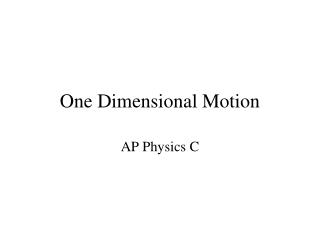# One Dimensional Motion - PowerPoint PPT PresentationDownload PresentationOne Dimensional Motion

One Dimensional MotionDownload Presentation## One Dimensional Motion

- - - - - - - - - - - - - - - - - - - - - - - - - - - E N D - - - - - - - - - - - - - - - - - - - - - - - - - - -
##### Presentation Transcript

1. One Dimensional Motion AP Physics C

2. Terms Displacement vs. Distance Displacement: Change in position (vector) • Distance: How far an object has travelled (scalar) • Symbol:Δx or Δx • SI Unit: meter, m

3. Position

4. Terms Velocity vs. Speed • Velocity: Rate of the change in position (vector) • Speed: Rate of distance covered (scalar) • Symbol:v or v • SI Unit: meter per second, m/s

5. Average Velocity

6. Instantaneous Velocity

7. Terms Acceleration vs. Acceleration • Acceleration: The rate at which velocity changes (vector) • Acceleration: The rate at which speed changes (scalar) • Symbol: a or a • SI Unit: meters per second per second or meters per second squared, m/s/s or m/s2

8. Average Acceleration

9. Instantaneous Acceleration

10. Position vs. Time Graph

11. What are the velocities?

12. What are the velocities?

13. Average Velocity on an x-t graph

14. vav vs vinst on an x-t graph

15. x-t graph vs motion of a particle

16. Instantaneous Velocity on an x-t graph

17. Velocity vs. Time Graph • The slope of a v vs. t graph is the acceleration • The area between the curve and the horizontal axis of a v vs. t graph is the displacement

18. Comparison of v-t to a-t graphs

19. v-t graph vs motion of a particle

20. x-t graph vs motion of a particle with acceleration

21. What are the accelerations and displacements?

22. What are the accelerations and displacements?

23. Acceleration vs. Time Graph • The slope means NOTHING • The area between the curve and the horizontal axis is the change in velocity a 10 s t -10 m/s/s

24. Important Acceleration tells us how fast velocity changes Velocity tells us how fast position changes

25. Kinematics Equations(accelerated motion)

26. Falling Bodies, thrown up objects, and the y-direction • All things fall at the same rate (neglecting air resistance) • On earth that rate is 9.80 m/s2 • That rate is an acceleration • The name of that acceleration is Gravity

27. Object moving in y-dir

28. Changing Acceleration

29. Velocity by Integration

30. Position by Integration